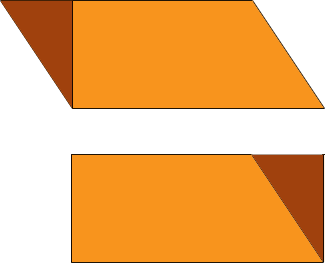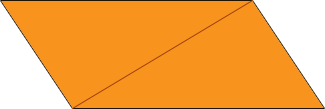SEARCH HOMEMath Central Quandaries & QueriesQuestion from Vivi, a student: Hi, my name is Vivi, and math teacher is giving a geometry test. I tend to forget how to find the surface area and volume of triangular pyramids and parallelograms. Do you have a way of remembering how to find the surface area?Hi,

I see two ways to view the area of a parallelogram that may make it easy to remember.

For the first method slice off dark region of the parallelogram below and slide it to the other end. Since the opposite sides are parallel the resulting figure will be a rectangle. Hence its area is the length of the base times the height and therefore the area of the parallelogram is the length of the base times the height where the height is the perpendicular distance from the base to the opposite side.For the second method draw a diagonal to divide the parallelogram into two congruent triangles. The area of each triangle is one half the base times the height so the area of the parallelogram is twice this, the length of the base times the height.The surface of a triangular pyramid is composed of four triangles, the base triangle and the three sides. To find the surface area you need to calculate the areas of each of the 4 triangles and add them. How you find the areas of the triangles depends on the information you are given about the pyramid.

Finding the volume of the pyramid is easier. What I remember is the volume of a cone, 1/3 π r2 h, but I remember is as

volume = 1/3 × (the area of the base) × (the height).

What is amazing is that this formula also works for a triangular pyramid and many other shapes. See the last paragraph of this item in our database.

HarleyMath Central is supported by the University of Regina and The Pacific Institute for the Mathematical Sciences.